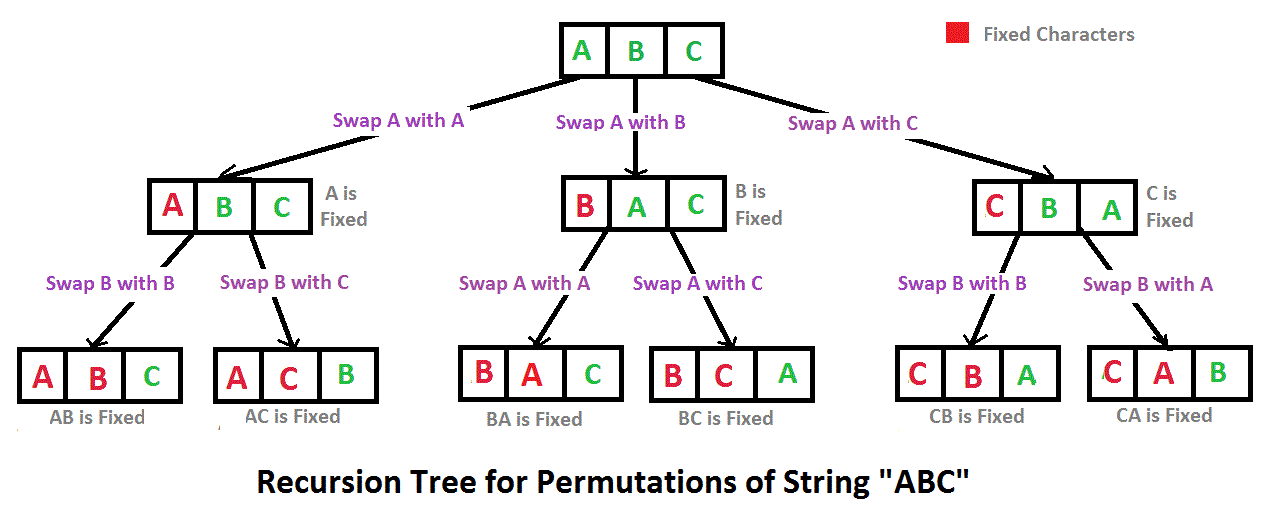# C# Program to print all permutations of a given string

• Difficulty Level : Hard
• Last Updated : 10 Dec, 2021

A permutation also called an “arrangement number” or “order,” is a rearrangement of the elements of an ordered list S into a one-to-one correspondence with S itself. A string of length n has n! permutation.

Source: Mathword(http://mathworld.wolfram.com/Permutation.html)

Below are the permutations of string ABC.
ABC ACB BAC BCA CBA CAB

Here is a solution that is used as a basis in backtracking.## C#

 `// C# program to print all permutations  ` `// of a given string.  ` `using` `System;  ` ` `  `class` `GFG  ` `{  ` `    ``/* Permutation function @param  ` `       ``str string to calculate permutation  ` `       ``for @param l starting index @param  ` `       ``r end index */` `    ``private` `static` `void` `permute(String str,  ` `                                ``int` `l, ``int` `r)  ` `    ``{  ` `        ``if` `(l == r)  ` `            ``Console.WriteLine(str);  ` `        ``else` `        ``{  ` `            ``for` `(``int` `i = l; i <= r; i++)  ` `            ``{  ` `                ``str = swap(str, l, i);  ` `                ``permute(str, l + 1, r);  ` `                ``str = swap(str, l, i);  ` `            ``}  ` `        ``}  ` `    ``}  ` ` `  `    ``/* Swap Characters at position  ` `       ``@param a string value @param  ` `       ``i position 1 @param j position 2  ` `       ``@return swapped string */` `    ``public` `static` `String swap(String a,  ` `                            ``int` `i, ``int` `j)  ` `    ``{  ` `        ``char` `temp;  ` `        ``char``[] charArray = a.ToCharArray();  ` `        ``temp = charArray[i] ;  ` `        ``charArray[i] = charArray[j];  ` `        ``charArray[j] = temp;  ` `        ``string` `s = ``new` `string``(charArray);  ` `        ``return` `s;  ` `    ``}  ` ` `  `// Driver Code  ` `public` `static` `void` `Main()  ` `{  ` `    ``String str = ``"ABC"``;  ` `    ``int` `n = str.Length;  ` `    ``permute(str, 0, n-1);  ` `}  ` `}  ` `// This code is contributed by mits  `

Output:

```ABC
ACB
BAC
BCA
CBA
CAB```

Time Complexity: O(n*n!) Note that there are n! permutations and it requires O(n) time to print a permutation.

Auxiliary Space: O(r – l)

Note: The above solution prints duplicate permutations if there are repeating characters in the input string. Please see the below link for a solution that prints only distinct permutations even if there are duplicates in input.
Print all distinct permutations of a given string with duplicates.
Permutations of a given string using STL

Another approach:

## C#

 `// C# program to implement ` `// the above approach ` `using` `System; ` `public` `class` `GFG{ ` `  `  `static` `void` `permute(String s, ` `                    ``String answer) ` `{    ` `    ``if` `(s.Length == 0) ` `    ``{ ` `        ``Console.Write(answer + ``"  "``); ` `        ``return``; ` `    ``} ` `     `  `    ``for``(``int` `i = 0 ;i < s.Length; i++) ` `    ``{ ` `        ``char` `ch = s[i]; ` `        ``String left_substr = s.Substring(0, i); ` `        ``String right_substr = s.Substring(i + 1); ` `        ``String rest = left_substr + right_substr; ` `        ``permute(rest, answer + ch); ` `    ``} ` `} ` ` `  `// Driver code ` `public` `static` `void` `Main(String []args) ` `{     ` `    ``String s; ` `    ``String answer=``""``; ` `     `  `    ``Console.Write( ` `    ``"Enter the string : "``); ` `    ``s = Console.ReadLine(); ` `     `  `    ``Console.Write( ` `    ``"\nAll possible strings are : "``); ` `    ``permute(s, answer); ` `} ` `} ` `// This code is contributed by gauravrajput1  `

Output:

```Enter the string : abc
All possible strings are : abc  acb  bac  bca  cab  cba```

Time Complexity: O(n*n!) The time complexity is the same as the above approach, i.e. there are n! permutations and it requires O(n) time to print a permutation.

Auxiliary Space: O(|s|)

Please refer complete article on Write a program to print all permutations of a given string for more details!

My Personal Notes arrow_drop_up
Recommended Articles
Page :